# GMAT Math : Calculating mode

## Example Questions

← Previous 1

### Example Question #1 : Mode

Rita keeps track of the number of times she goes to the gym each week for 1260 weeks.  She goes 1 day a week for 119 weeks, 2 days a week for 254 weeks, 3 days a week for 376 weeks, and 4 days a week for 511 weeks.  What is the mode of the number of days she goes to the gym each week?

119 weeks

4 days/week

1 day/week

2.5 days/week

511 weeks

4 days/week

Explanation:

The mode is the number that comes up most frequently in a set.  Rita goes to the gym 4 times a week for 511 weeks.  She clearly goes 4 times per week far more often than she goes 1, 2, or 3 times per week.  Therefore the mode is 4 days/week.  It is NOT 511 weeks.  That is the frequency with which 4 days/week occurs, but not the mode.

### Example Question #2101 : Gmat Quantitative Reasoning

For which of the following values ofwould the median and the mode of the data set be equal?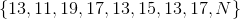None of the other answers are correct.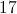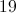Explanation:

If the known values are ordered from least to greatest, the set looks like this: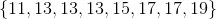Below are each of the choices, followed by the set that results if it is added to the above set, followed by the median - the middle element - and the mode - the most frequently occurring element.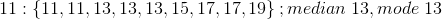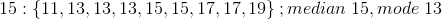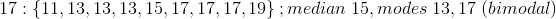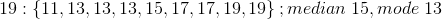Only the addition of 11 yields a set with median and mode equal to each other.

### Example Question #2102 : Gmat Quantitative Reasoning

Consider the data set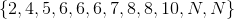. It is known that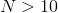. How many modes does this data set have, and what are they?

The set has one mode, 6.

The set has one mode,.

The set has two modes, 6 and.

The set has three modes, 6, 8, and.

The set has two modes, 6 and 8.

The set has one mode, 6.

Explanation:

Of the known elements, 6 occurs the most frequently - three times. Since the unknownoccurs only twice, and it cannot be equal to any of the other elements, its value does not affect the status of 6 as the most frequent element. Therefore, regardless of, 6 is the only mode.

### Example Question #94 : Descriptive Statistics

What is the mode for the following set: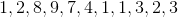Explanation:

The mode is the number that appears most frequently:### Example Question #552 : Arithmetic

Determine the mode of the following set of data: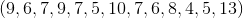Explanation:

The mode of a set of data is the entry that appears most often within the set. One easy way to determine the mode is by arranging the set in increasing order: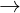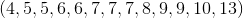Now that the set is arranged in increasing order, we can see how often each value appears in the set. The value 7 appears three times, which is more than any other entry is repeated, so this is the mode of the set.

### Example Question #553 : Arithmetic

Determine the mode of the following set of data: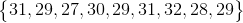Explanation:

The mode of a set of data is the entry that occurs most often within the set. An easy way to determine which entry occurs the most often is by arranging the set in increasing order: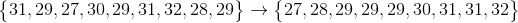Now we can see that 29 is repeated more often than any other number in the set, so this is the mode.

### Example Question #554 : Arithmetic

Determine the mode of the following set of data: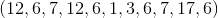Explanation:

The mode of a set of data is the entry that appears most frequently within the set. An easy way to determine the mode is by arranging the set in increasing order: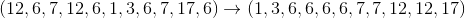Now we can see that the value ofis repeated more times than any other value, so this is the mode of the set of data.

### Example Question #101 : Descriptive Statistics

Determine the mode for the following set of numbers.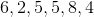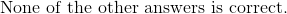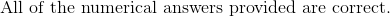Explanation:

The mode is the most frequent number, thus our answer is.

### Example Question #2 : Calculating Mode

Find the mode of the following set of numbers: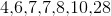Explanation:

The mode is the most frequent number, thus the answer is.

### Example Question #557 : Arithmetic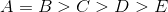Give the mode of the set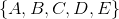.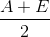The set has no mode.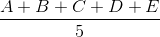Explanation:

The mode of a set, if it exists, is the value that occurs most frequently. The inequalitymeans that the setcan be rewritten as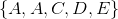.

The most frequently occurring value is, making this the mode.

← Previous 1

Tired of practice problems?

Try live online GMAT Math prep today.